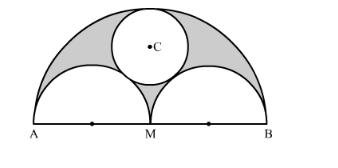# In the following figure, AB = 36 cm and M is mid-point of AB.Question:

In the following figure, AB = 36 cm and M is mid-point of AB. Semi-circles are drawn on ABAM and MB as diameters. A circle with centre C touches all the three circles. Find the area of the shaded region.Solution:

We have given two semi-circles and one circle.

Area of the shaded region = area of semicircle with diameter AB − area of two semicircles with diameters AM and MB - area of circle ……..(1)

Let us calculate the area of the semi-circle with AB as a diameter.

Area of semi-circle with $\mathrm{AB}$ as a diameter $=\frac{\pi r^{2}}{2}$

$\therefore$ Area of semi-circle with $\mathrm{AB}$ as a diameter $=\frac{\pi\left(\frac{36}{2}\right)^{2}}{2}$

$\therefore$ Area of semi-circle with $\mathrm{AB}$ as a diameter $=\frac{\pi \times 18^{2}}{2}$

Now we will find the area of the semi-circle with AM as a diameter.

Area of semi-circle with AM as a diameter $=\frac{\pi r^{2}}{2}$

$\therefore$ Area of semi-circle with AM as a diameter $=\frac{\pi\left(\frac{18}{2}\right)^{2}}{2}$

$\therefore$ Area of semi-circle with AB as a diameter $=\frac{\pi \times 9^{2}}{2}$

Area of the semi-circle with MB as a diameter is same as the area of the semi-circle with diameter with AM as a diameter.

Now we will find the area of the circle with centre C.

Area of circle $=\pi r^{2}$

We know that radius of the circle is one sixth of AB.

Area of circle $=\pi \times 6^{2}$

Now we will substitute all these values in equation (1).

$\therefore$ Area of shaded region $=\frac{\pi \times 18^{2}}{2}-\frac{\pi \times 9^{2}}{2}-\frac{\pi \times 9^{2}}{2}-36 \pi$

$\therefore$ Area of shaded region $=\frac{\pi \times 18^{2}}{2}-\pi \times 9^{2}-36 \pi$

$\therefore$ Area of shaded region $=\frac{\pi \times 18^{2}}{2}-81 \pi-36 \pi$

$\therefore$ Area of shaded region $=\frac{\pi \times 18^{2}}{2}-117 \pi$

$\therefore$ Area of shaded region $=(162-117) \pi$

$\therefore$ Area of shaded region $=45 \pi$

Therefore, area of shaded region is $45 \pi \mathrm{cm}^{2}$